# Creating Polygon Shapes

2 May 2017Nazca Layout

# Creating Polygon Shapes

### How to place polygons and use predefined Nazca shapes

```# How to make geometric shapes using polygons
import nazca as nd
import nazca.geometries as geom

frame = nd.Polygon(layer=19, points=[(0,0), (220,0), (220,180), (0,180)])
boat = nd.Polygon(layer=56, points=[(0,0), (120,0), (130,40), (-10, 40)])
sail = nd.Polygon(layer=52, points=[(0,0), (100,0), (90,100)])

# position the polygons
frame.put(-10, 0, 0)
boat.put(40, 20, 0)
sail.put(50, 70, -10)
sun.put(40,110)
hole.put(10,160,0)
hole.put(190,160,0)

nd.export_gds(filename='DickBruna.gds')```

Custom building blocks may require implementation of specific shapes, typically made by a list of points. In this example we show how to place polygons in different layers and use predefined shapes from the nazca.geometries module. As an example, we take Dick Bruna’s sailing boat.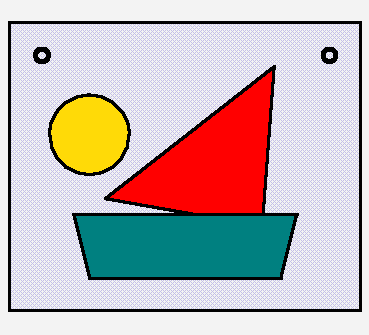## Related Tutorials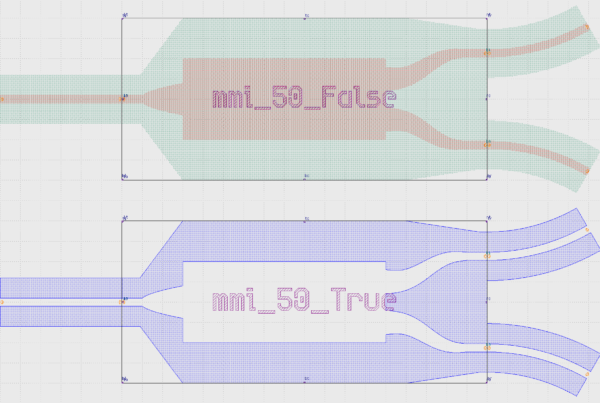In this example we show how to create an inverted MMI with custom interconnects.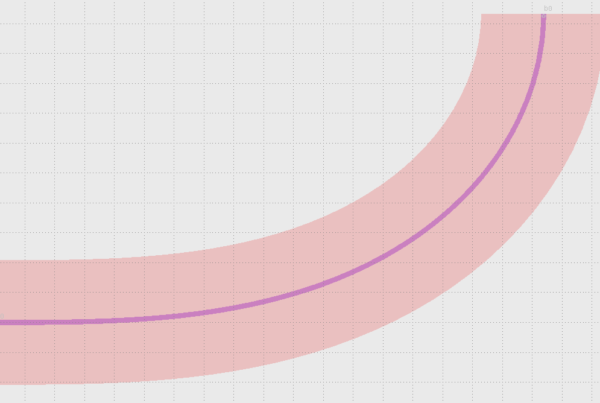In this example we show how to use Euler bends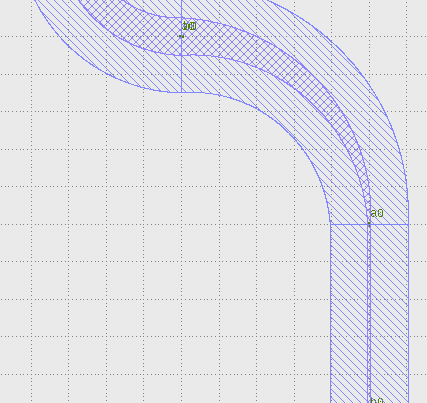In this example we show how to create a free form parametric curve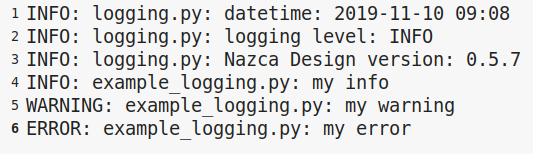In this example we show how to log your layout.